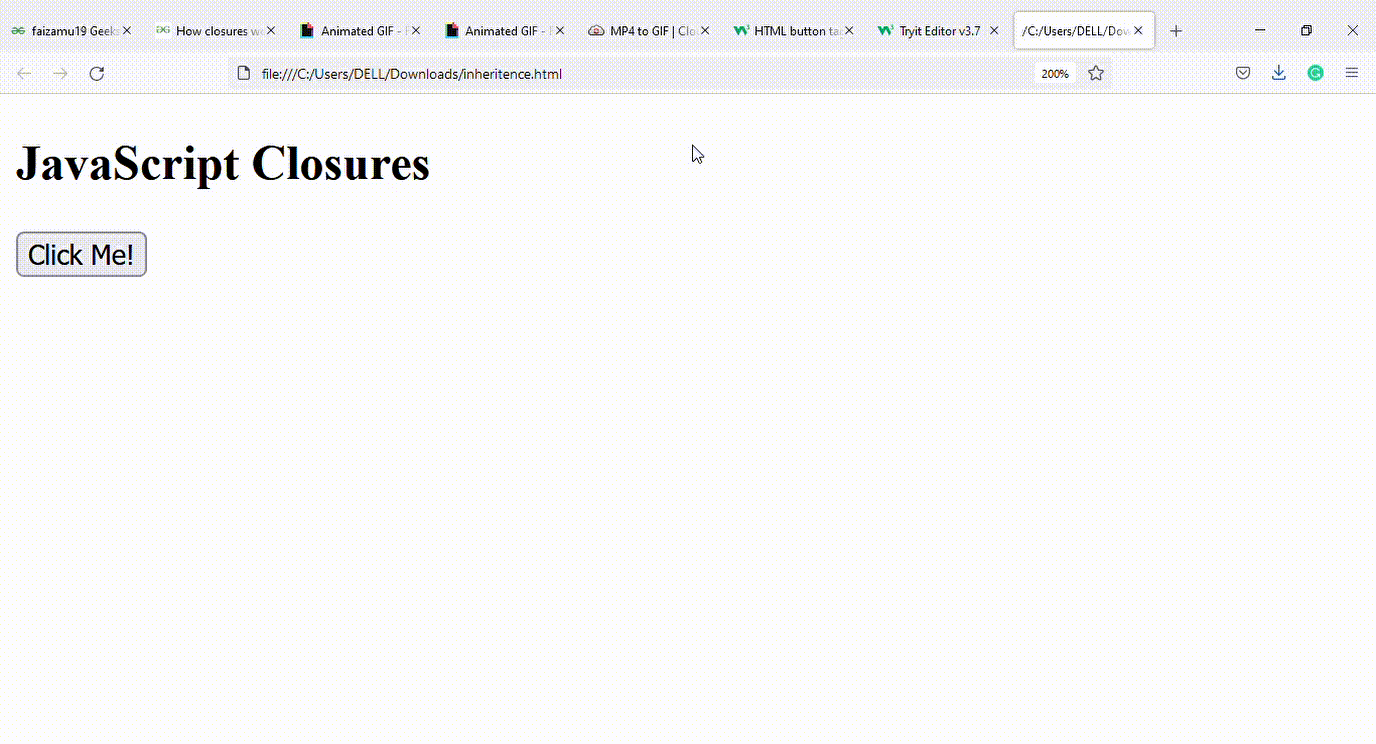Open in App
Not now

# How closure works in JavaScript ?

• Last Updated : 23 Nov, 2021

In this article, we will discuss about the closures working JavaScript. Let us first understand what exactly closures are and basic details which are associated with closures in JavaScript.

A Closure is a combination of a function enclosed with references to its surrounding state (the lexical environment). In JavaScript, closures are created every time a function is created at run time. In other words, a closure is just a fancy name for a function that remembers the external things used inside it.

Let us look at some examples to understand how closures actually work in JavaScript.

Example 1:

• In this example, we will be declaring a closure which would eventually access an outer variable balance from the outer function.
• After using the outer variable in inner most function, that particular closure will help us to deduct 100 from it, each time whenever that outer function is called.

## HTML

 ` ` `<``html``> ` ` `  `<``body``> ` `    ``<``h2``>JavaScript Closures ` `    ``<``button` `type``=``"button"` `onclick``=``"initaccount()"``> ` `          ``Click Me! ` `        `` ` `    ``<``p` `id``=``"demo"``> ` `    ``<``script``> ` `    ``function initaccount() { ` `        ``var balance = 1000; ` ` `  `        ``function currentbalance() { ` `            ``balance = balance - 100; ` `            ``alert(balance); ` `        ``} ` `        ``currentbalance(); ` `    ``} ` `    `` ` ` ` ` `  ` `

Output:Explanation: In the above example, currentbalance() can access outer variable balance hence balance is deducted by 100 each time initaccount() method is called.

Example 2: Closures can be nested as well as in below example. Here in the example both outerfunction() and innerfunction() has access to counter variable , hence on calling Counter() both outerfunction() and innerfunction() increments the value of counter. In this context, we can say that closures have access to all outer function scopes.

## HTML

 ` ` `<``html``> ` ` `  `<``body``> ` `    ``<``h2``>JavaScript Closures ` `    ``<``button` `type``=``"button"` `onclick``=``" Counter()"``> ` `        ``Click Me! ` `    `` ` `    ``<``p` `id``=``"demo1"``> ` `    ``<``p` `id``=``"demo2"``> ` `    ``<``script``> ` `    ``function Counter() { ` `        ``var counter = 0; ` ` `  `        ``function outerfunction() { ` `            ``counter += 1; ` `            ``document.getElementById("demo1").innerHTML ` `                ``= "outercounter = " + counter + ` `                ``"from outerfunction " ; ` ` `  `            ``function innerfunction() { ` `                ``counter += 1; ` `                ``document.getElementById("demo2").innerHTML ` `                 ``= " innercounter = " + counter +  ` `                   ``"from innerfunction "; ` `            ``}; ` `            ``innerfunction(); ` `        ``}; ` `        ``outerfunction(); ` `    ``}; ` `    `` ` ` ` ` `  ` `

Output:My Personal Notes arrow_drop_up
Related Articles